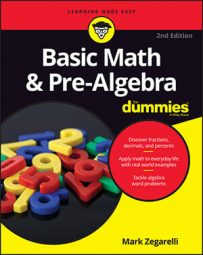##### Basic Math & Pre-Algebra For DummiesThe Big Four math operations — addition, subtraction, multiplication, and division — let you combine numbers and perform calculations. Certain operations possess properties that enable you to manipulate the numbers in the problem, which comes in handy, especially when you get into higher math like algebra. The important properties you need to know are the commutative property, the associative property, and the distributive property. Understanding what an inverse operation is is also helpful.

## Inverse operations

Inverse operations are pairs of operations that you can work "backward" to cancel each other out. Two pairs of the Big Four operations — addition, subtraction, multiplication, and division —are inverses of each other:
• Addition and subtraction are inverse operations of each other. When you start with any value, then add a number to it and subtract the same number from the result, the value you started with remains unchanged. For example:

2 + 3 = 5 so 5 – 3 = 2
7 – 1 = 6 so 6 + 1 = 7
• Multiplication and division are inverse operations of each other. When you start with any value, then multiply it by a number and divide the result by the same number (except zero), the value you started with remains unchanged. For example:

3 × 4 = 12 so 12 ÷ 4 = 3
10 ÷ 2 = 5 so 5 × 2 = 10

## The commutative property

An operation is commutative when you apply it to a pair of numbers either forwards or backwards and expect the same result. The two Big Four that are commutative are addition and subtraction.

Addition is commutative because, for example, 3 + 5 is the same as 5 + 3. In other words

3 + 5 = 5 + 3
Multiplication is commutative because 2 × 7 is the same as 7 × 2. In other words
2 × 7 = 7 × 2

## The associative property

An operation is associative when you can apply it, using parentheses, in different groupings of numbers and still expect the same result. The two Big Four operations that are associative are addition and multiplication.

Addition is associative because, for example, the problem (2 + 4) + 7 produces the same result as does the problem 2 + (4 + 7). In other words,

(2 + 4) + 7 = 2 + (4 + 7)
No matter which pair of numbers you add together first, the answer is the same: 13.

Multiplication is associative because, for example, the problem 3 × (4 × 5) produces the same result as the problem (3 × 4) × 5. In other words,

3 × (4 × 5) = (3 × 4) × 5
Again, no matter which pair of numbers you multiply first, both problems yield the same answer: 60.

## The distributive property

The distributive property connects the operations of multiplication and addition. When multiplication is described as "distributive over addition," you can split a multiplication problem into two smaller problems and then add the results.

For example, suppose you want to multiply 27 × 6. You know that 27 equals 20 + 7, so you can do this multiplication in two steps:

1. First multiply 20 × 6; then multiply 7 × 6.

20 × 6 = 1207 × 6 = 42

120 + 42 = 162

Therefore, 27 × 6 = 162.